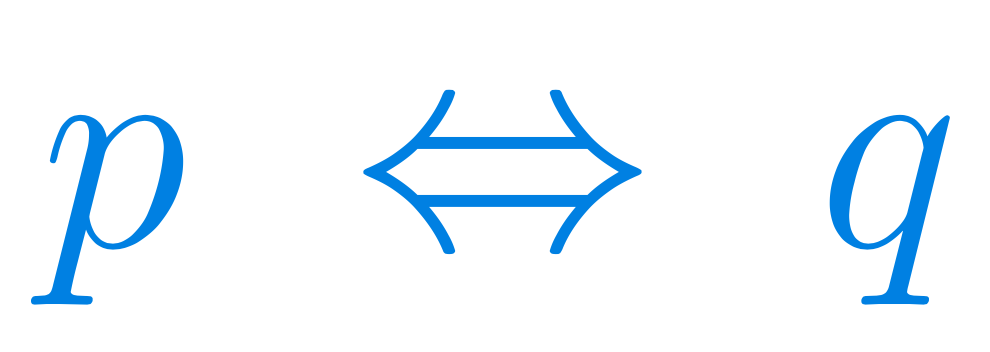Select Page

# Precalculus 1: basic notions

#### A total of 52 hours of lectures

Due to the size of precalculus, it is divided into four parts. This page describes the first of those four parts.#### Prerequisites

High-school maths, mainly arithmetics, some trigonometry

#### Curriculum

Make sure that you check with your professor what parts of the course you will need for your exam. Such things vary from country to country, from university to university, and they can even vary from year to year at the same university.

# Precalculus: basic notions

h

## Get the outline

A detailed list of all the lectures in part 1 of the course, including which theorems will be discussed and which problems will be solved. If you are looking for a particular kind of problem or a particular concept, this is where you should look first.## Get Precalculus 1: basic notions on Udemy

When you buy the course on Udemy, you get access to it for life. There is just a one-time fee. The prices do vary a lot on Udemy, but if you use our link by clicking on this panel, you will get the best current price. See also our page on “coupon codes” in the menu (the current code is TPOT_DEC23).

## Course Objectives & Outcomes

Z
How to solve problems in chosen Precalculus topics (illustrated with 236 solved problems) and why these methods work.
Z
The basic notions will be defined in a more abstract way, and you will get an insight into their place in Calculus and some other branches of university maths.
Z
Arithmetic with basic rules (associativity and commutativity of addition and multiplication, distributivity) illustrated for positive integers.
Z
Basic concepts related to functions (domain, range, graph, surjection, injection, bijection, inverse, composition) and how to work with them.
Z
Geometrical operations on graphs of functions: translations, reflections, shrinking, dilating. Piecewise functions.
Z
Equations of straight lines in the plane: slope and intercept; first glimpse into derivative as the slope of the tangent line, and link to monotonicity.
Z
Logic: symbols and rules (tautologies) used for creating mathematical statements, definitions, and proofs.
Z
Relations: RST (equivalence) relations, order relations, functions as relations.
Z
Various types of proofs: direct, indirect, by contradiction, induction proof, with some examples.
Z
Decimal expansion of rational and irrational numbers. Density of Q and R\Q in R.
Z
You will also get a solid background for any future studies in Real Analysis and other courses in university mathematics.
Z
Repetition of chosen aspects of high school mathematics (basic notions as numbers, functions, sets, equations, and inequalities).
Z
By the end of this course you will be able to understand all the elements in the epsilon-delta definition of limit, both symbols and the content.
Z
Basic terminology for equations and inequalities, with examples of linear equations and inequalities, with and without absolute value.
Z
Functions: monotone (increasing, decreasing), bounded, even, odd; extremum: maximum, minimum, both local and global.
Z
Absolute value and its role in computing distances, with geometrical illustrations and functional approach.
Z
Introduction to sequences and series; short notation for sum (Sigma) and product (Pi); arithmetic, geometric, and harmonic progressions.
Z
Set theory: union, intersection, complement, set difference; some important rules and notation.
Z
Necessary and sufficient conditions: definition and examples.
Z
Building blocks of mathematical theories: axioms, definitions, theorems, propositions, lemmas, corollaries, etc.
Z
Cardinality of sets: finite sets, N, Z, Q, R; countable and uncountable sets.